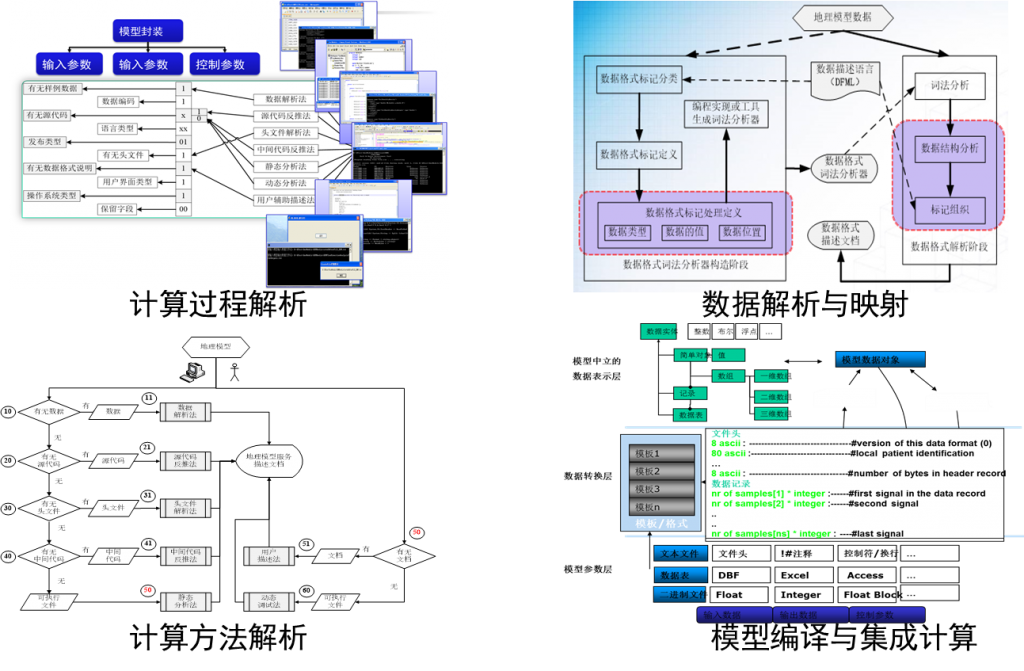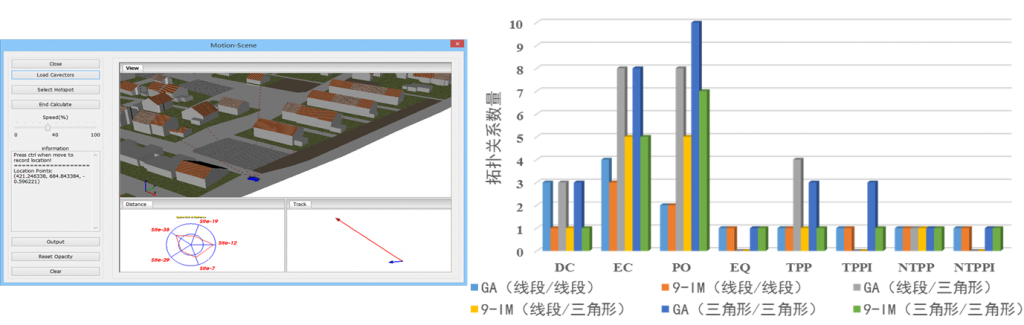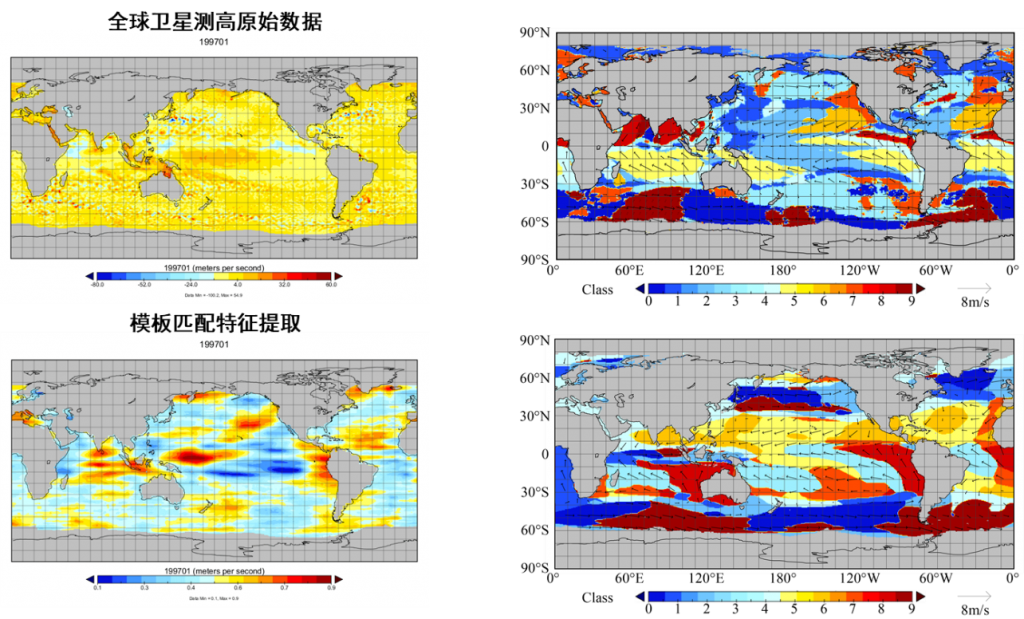### Adaptive integration and transformation of geographic models

In terms of data expression, integration and updating, using algebraic and symbolic representation of what broke the existing gap between all kinds of GIS data, set up the mathematical expression of different kinds of GIS data, implement the data fusion expression, characteristics, relationship and support geometric algebra calculation. Based on the geometric algebraic operator algorithm, the translation, transformation and construction of the geographic analysis model are realized through the analysis of the model calculation grid and calculation process. At the computational level of the model, the calculation of the computation of the code on the heterogeneous platform by the operator and the template is realized automatically and optimized.### Embedding of GIS dynamic models：dynamic topological relation computation model

Topology relationship computing operator is constructed, which breaks the problem of continuous dynamic calculation of topological relation. From the perspective of dynamic view, spatio-temporal process analysis and geographic model integration, the GIS analysis method and the support of geographical model are explored. In the dynamic topological relations, the existing topology relationship analysis mainly based on set theory Angle of view, on the basis of the qualitative interpretation of quantitative calculation, and we set off from the perspectives of geometric algebra, the dimension calculation and calculation on the basis of the relationship between, developed algebra, the formalization of spatial topological relations between expression and calculation method, constructs the model for calculating the dynamic spatial relations.### Embedding of structural feature models: spatio-temporal feature analysis of high dimensional data

The uniform expression and calculation of scalar and vector, characteristic and structure, process and parameter are realized. In high dimensional space and time features of the data analysis method, the independent application in the low dimensional scalar field or vector field of differential, convolution method is extended to high dimension, realized the scalar field, vector field and the unity of the multiple vector field analysis, and from the perspective of expression and characteristics of space-time process developed based on the scalar field of time and space of geometric algebra, vector field of time and space, and the multiple characteristic parameters and structure of the vector field of time and space analysis method.### Embedding of continuous geographic models: integration of continuous multi-scale geographic model .

Developed both spatial and temporal data management and model calculation of geometric algebra coding method, multi-scale model integration aspects of geography, GIS and discrete model calculation focused on grid, but GIS is more focused on the geometric structure, and the model calculation is more concerned with relationship expressed by the grid structure; This kind of inconsistency leads to the difficulties in the integration of GIS and pattern calculation. Based on geometric algebraic multidimensional object hierarchical structure relation, and the unity of geometric expression and algebraic operation, we developed geometric algebraic coding method for spatio-temporal data management and model computation.### Fusion construction multi-element scenario

Integrated modeling and simulation of emergency evacuation for geographic scene constraints. With GA city modeling, data transformation and the emergency evacuation of the case of integrated modeling and simulation, based on the geometric algebra scene model can realize the scenario of different kinds of data integration modeling and through dynamic updating model, and can effectively simplify the dynamic coupling model of the complexity of the dynamic update of data and calculation.